##### The Japanese Bonsai specialist
Direct order Contact Help / Services Newsletter# Mini bonsai ilex, malus and fruit handbook n°5

Kyosuke gun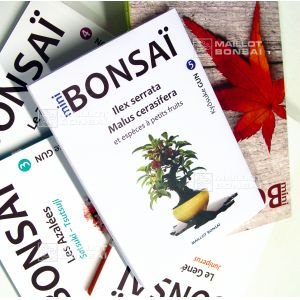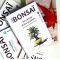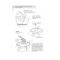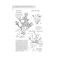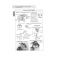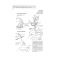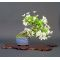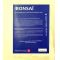ref. : 3219

24,00

Available quantity : 59Order

###### Description

ISBN: 9782953428247

Author: Mr Kyosuke

Format: 144 pages, 17*25 cm

Manga style (read from back to front)

Translated from Japanese into French

This practical 'how to' bonsai book tells you all you need to know about ilex serrata, malus sieboldii miyama kaido and malus cerasifera. There is currently no English version but easy to understand, clear diagrams and pictures demonstrate how to care for your fruit-bearing bonsai throughout the seasons. There are tips on fruit growth, watering, fertilisation, pollination, seed collection, repotting and creating different bonsai forms.

An essential technical handbook for mini bonsai. The same techniques apply to large bonsai.

#bonsai 7.6 #malus 4.5 #fruit 4.5 #handbook 3.8 #ilex 3.4 #mini 3.4 #there 2.5 #books 2.5 #from 2.4 #fertilisation 2.3

Formule
(( ROUND((CHAR_LENGTH(b.article_nom)-CHAR_LENGTH(REPLACE(b.article_nom, 'bonsai', '')))/LENGTH('bonsai')) + ROUND((CHAR_LENGTH(b.article_description)-CHAR_LENGTH(REPLACE(b.article_description, 'bonsai', '')))/LENGTH('bonsai')) ) * 7.6) + (( ROUND((CHAR_LENGTH(b.article_nom)-CHAR_LENGTH(REPLACE(b.article_nom, 'malus', '')))/LENGTH('malus')) + ROUND((CHAR_LENGTH(b.article_description)-CHAR_LENGTH(REPLACE(b.article_description, 'malus', '')))/LENGTH('malus')) ) * 4.5) + (( ROUND((CHAR_LENGTH(b.article_nom)-CHAR_LENGTH(REPLACE(b.article_nom, 'fruit', '')))/LENGTH('fruit')) + ROUND((CHAR_LENGTH(b.article_description)-CHAR_LENGTH(REPLACE(b.article_description, 'fruit', '')))/LENGTH('fruit')) ) * 4.5) + (( ROUND((CHAR_LENGTH(b.article_nom)-CHAR_LENGTH(REPLACE(b.article_nom, 'handbook', '')))/LENGTH('handbook')) + ROUND((CHAR_LENGTH(b.article_description)-CHAR_LENGTH(REPLACE(b.article_description, 'handbook', '')))/LENGTH('handbook')) ) * 3.8) + (( ROUND((CHAR_LENGTH(b.article_nom)-CHAR_LENGTH(REPLACE(b.article_nom, 'ilex', '')))/LENGTH('ilex')) + ROUND((CHAR_LENGTH(b.article_description)-CHAR_LENGTH(REPLACE(b.article_description, 'ilex', '')))/LENGTH('ilex')) ) * 3.4) + (( ROUND((CHAR_LENGTH(b.article_nom)-CHAR_LENGTH(REPLACE(b.article_nom, 'mini', '')))/LENGTH('mini')) + ROUND((CHAR_LENGTH(b.article_description)-CHAR_LENGTH(REPLACE(b.article_description, 'mini', '')))/LENGTH('mini')) ) * 3.4) + (( ROUND((CHAR_LENGTH(b.article_nom)-CHAR_LENGTH(REPLACE(b.article_nom, 'there', '')))/LENGTH('there')) + ROUND((CHAR_LENGTH(b.article_description)-CHAR_LENGTH(REPLACE(b.article_description, 'there', '')))/LENGTH('there')) ) * 2.5) + (( ROUND((CHAR_LENGTH(b.article_nom)-CHAR_LENGTH(REPLACE(b.article_nom, 'from', '')))/LENGTH('from')) + ROUND((CHAR_LENGTH(b.article_description)-CHAR_LENGTH(REPLACE(b.article_description, 'from', '')))/LENGTH('from')) ) * 2.4) + (( ROUND((CHAR_LENGTH(b.article_nom)-CHAR_LENGTH(REPLACE(b.article_nom, 'fertilisation', '')))/LENGTH('fertilisation')) + ROUND((CHAR_LENGTH(b.article_description)-CHAR_LENGTH(REPLACE(b.article_description, 'fertilisation', '')))/LENGTH('fertilisation')) ) * 2.3) + (( ROUND((CHAR_LENGTH(b.article_nom)-CHAR_LENGTH(REPLACE(b.article_nom, 'pollination', '')))/LENGTH('pollination')) + ROUND((CHAR_LENGTH(b.article_description)-CHAR_LENGTH(REPLACE(b.article_description, 'pollination', '')))/LENGTH('pollination')) ) * 2.1)

## Secure payment## Delivery

Our logistic partners :